#### 期刊菜单

A General Software Phase-Locked Loop Compatible with Single-Phase System
DOI: 10.12677/JEE.2022.101002, PDF , HTML, XML, 下载: 196  浏览: 325

Abstract: When the high-power onboard charger works as V2G mode, there will be an application scenario of single-phase three-phase system switching. In this paper, a general three-phase system phase- locked loop control method is proposed. This method regards the single-phase system as a special case of three-phase unbalanced fault, and realizes the positive and negative sequence separation of the voltage signal based on the phase-shift control method. The universal software phase- locked loop makes it not only suitable for three-phase systems, but also compatible with single- phase systems. Finally, through model simulation, for the application scenarios of three-phase voltage unbalance and single-phase three-phase system switching, the simulation verifies the correctness of the phase-locked loop design.

1. 引言

2. 单相电压的同步旋转坐标等效模型分析

2.1. 单相电压等效为三相不平衡故障的电压模型

$\left\{\begin{array}{l}{u}_{a}\left(t\right)=A\mathrm{sin}\left(\omega t+\phi \right)\hfill \\ {u}_{b}\left(t\right)=0\hfill \\ {u}_{c}\left(t\right)=0\hfill \end{array}$ (1)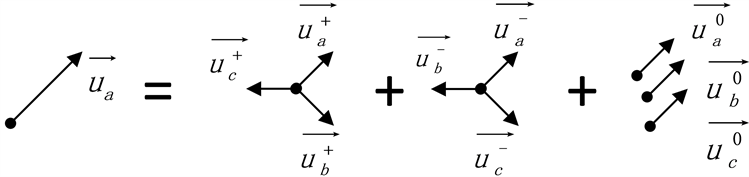Figure 1. Three-phase unbalanced voltage model of single-phase grid voltage

$\left\{\begin{array}{l}{u}_{a}={u}_{a}^{+}{\text{e}}^{j0˚}+{u}_{a}^{-}{\text{e}}^{j0˚}+{u}_{a}^{0}{\text{e}}^{j0˚}\\ 0={u}_{a}^{+}{\text{e}}^{j240˚}+{u}_{a}^{-}{\text{e}}^{j120˚}+{u}_{a}^{0}{\text{e}}^{j0˚}\\ 0={u}_{a}^{+}{\text{e}}^{j120˚}+{u}_{a}^{-}{\text{e}}^{j240˚}+{u}_{a}^{0}{\text{e}}^{j0˚}\end{array}$ (2)

${u}_{a}^{+}={u}_{a}^{-}={u}_{a}^{0}=\frac{A}{3}$ (3)

$\left[\begin{array}{c}{u}_{a}\\ 0\\ 0\end{array}\right]=\frac{A}{3}\left[\begin{array}{c}{\text{e}}^{j0˚}\\ {\text{e}}^{j240˚}\\ {\text{e}}^{j120˚}\end{array}\right]+\frac{A}{3}\left[\begin{array}{c}{\text{e}}^{j0˚}\\ {\text{e}}^{j120˚}\\ {\text{e}}^{j240˚}\end{array}\right]+\frac{A}{3}\left[\begin{array}{c}{\text{e}}^{j0˚}\\ {\text{e}}^{j0˚}\\ {\text{e}}^{j0˚}\end{array}\right]$ (4)

2.2. 双同步旋转坐标系下的特性分析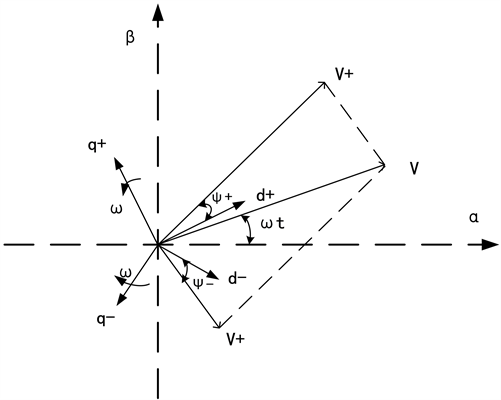Figure 2. Double synchronous rotating coordinate

$\left[\begin{array}{c}{V}_{d}^{+}\\ {V}_{q}^{+}\end{array}\right]=\frac{A}{3}\left[\begin{array}{c}\mathrm{cos}\left(\phi \right)\\ \mathrm{sin}\left(\phi \right)\end{array}\right]+\left[\begin{array}{cc}\mathrm{cos}\left(-2\omega t\right)& -\mathrm{sin}\left(-2\omega t\right)\\ \mathrm{sin}\left(-2\omega t\right)& \mathrm{cos}\left(-2\omega t\right)\end{array}\right]×\frac{A}{3}\left[\begin{array}{c}\mathrm{cos}\left(-\phi \right)\\ \mathrm{sin}\left(-\phi \right)\end{array}\right]$ (5)

$\left[\begin{array}{c}{V}_{d}^{-}\\ {V}_{q}^{-}\end{array}\right]=\frac{A}{3}\left[\begin{array}{c}\mathrm{cos}\left(-\phi \right)\\ \mathrm{sin}\left(-\phi \right)\end{array}\right]+\left[\begin{array}{cc}\mathrm{cos}\left(2\omega t\right)& -\mathrm{sin}\left(2\omega t\right)\\ \mathrm{sin}\left(2\omega t\right)& \mathrm{cos}\left(2\omega t\right)\end{array}\right]×\frac{A}{3}\left[\begin{array}{c}\mathrm{cos}\left(\phi \right)\\ \mathrm{sin}\left(\phi \right)\end{array}\right]$ (6)

3. 兼容单相系统的通用型锁相环控制设计

3.1. 移相控制器实现正负序分离

$\left\{\begin{array}{l}{u}_{d}={U}^{+}\mathrm{cos}{\phi }^{+}+{U}^{-}\mathrm{cos}\left(2{\omega }_{0}t+{\phi }^{-}\right)\\ {u}_{q}={U}^{+}\mathrm{sin}{\phi }^{+}-{U}^{-}\mathrm{sin}\left(2{\omega }_{0}t+{\phi }^{-}\right)\end{array}$ (7)

${u}_{d}$${u}_{q}$ 相移90˚，得到：

$\left\{\begin{array}{l}\stackrel{¯}{{u}_{d}}={U}^{+}\mathrm{cos}{\phi }^{+}+{U}^{-}\mathrm{sin}\left(2{\omega }_{0}t+{\phi }^{-}\right)\\ \stackrel{¯}{{u}_{q}}={U}^{+}\mathrm{sin}{\phi }^{+}+{U}^{-}\mathrm{cos}\left(2{\omega }_{0}t+{\phi }^{-}\right)\end{array}$ (8)

$\left\{\begin{array}{l}{u}_{d}^{+}={U}^{+}\mathrm{cos}{\phi }^{+}=0.5\left({u}_{d}+{u}_{q}+\stackrel{¯}{{u}_{d}}-\stackrel{¯}{{u}_{q}}\right)\\ {u}_{q}^{+}={U}^{+}\mathrm{sin}{\phi }^{+}=0.5\left(-{u}_{d}+{u}_{q}+\stackrel{¯}{{u}_{d}}+\stackrel{¯}{{u}_{q}}\right)\end{array}$ (9)

3.2. 移相控制器

$\left\{\begin{array}{l}\mathrm{cos}2\omega t+\mathrm{cos}2\omega \left(t-\frac{T}{4}\right)=0\\ \mathrm{sin}2\omega t+\mathrm{sin}2\omega \left(t-\frac{T}{4}\right)=0\end{array}$ (10)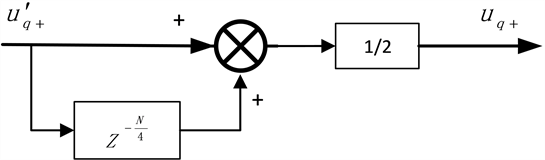Figure 3. Discrete block diagram of a phase-shift controller

3.3. 通用性锁相环控制框图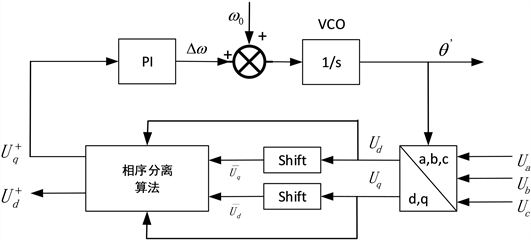Figure 4. Control block of phase-locked loop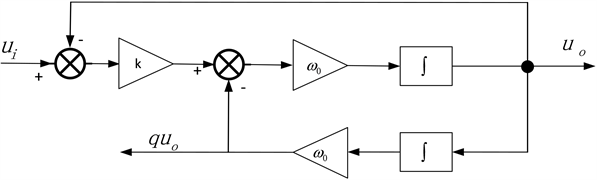Figure 5. Phase-shift control based on second-order generalized integrator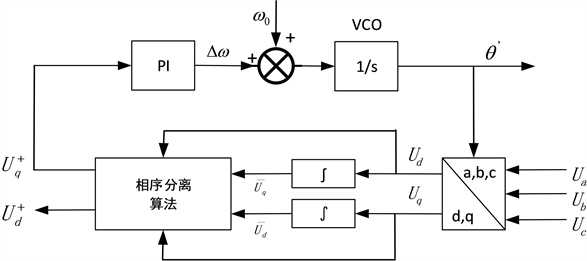Figure 6. Software phase locked loop based on second order generalized integrator

4. 仿真与实验效果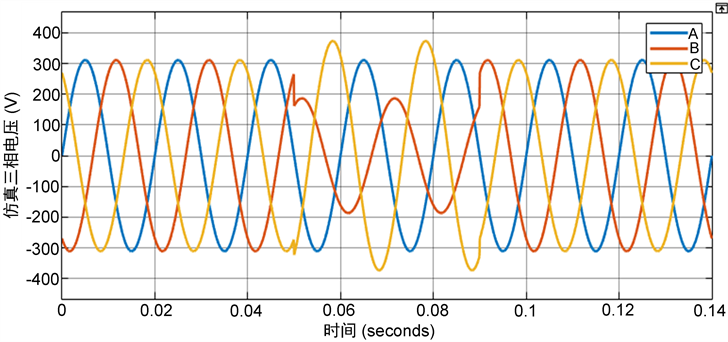Figure 7. The simulated three-phase voltage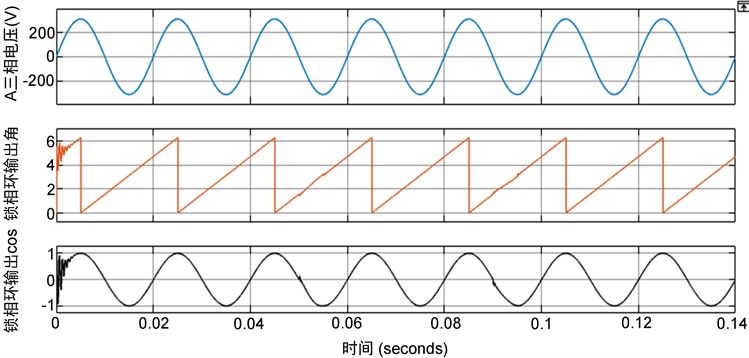Figure 8. The output of the phase-locked loop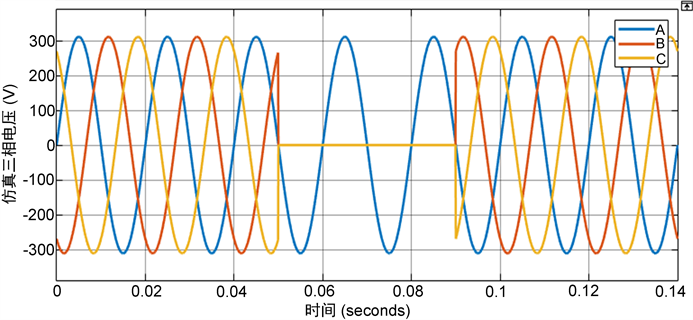Figure 9. Single-phase three-phase voltage switching simulation waveformFigure 10. Phase-locked loop output when single-phase three-phase voltage switching

5. 结论

  张兴, 张崇巍. PWM整流器机器控制[M]. 北京: 机械工业出版社, 2012: 411-412.  张学广, 徐殿国, 李伟伟. 一种适用于电网不平衡故障情况的数字锁相环[J]. 电气应用, 2009, 28(6): 54-58.  张振波, 王海云, 王维庆, 等. 基于改进型环路滤波器的单相锁相环[J]. 电力系统保护与控制, 2021, 49(13): 135-141.  马先进, 马家庆. 电网电压不平衡时高阶锁相环特性仿真研究[J]. 微处理机, 2021(6): 52-55.  潘志锋, 王孝洪, 吴春台, 等. 基于分数阶PID控制器和滑动平均滤波器的三相锁相环[J]. 高电压技术, 2021(11): 1-10.  周科, 刘伯鸿, 高峰阳, 等. 改进型双二阶广义积分器锁相环[J]. 电测与仪表, 2019, 56(17): 135-138.  杨仁增, 张良, 李鑫海, 等. 弱电网条件下的单相频率自适应锁相环[J]. 机械与电子, 2021, 39(9): 12-15.  朱建红, 顾菊平, 孟棒棒, 等. 软硬锁相环在分布式风电并网控制中的联合应用[J]. 太阳能学报, 2022, 43(1): 36-43.  Karimi-Ghartemani, M. AND Iravani, M.R. (2004) A Method for Synchronization of Power Electronic Converters in Polluted and Variable Frequency Environment. IEEE Transactions on Power Systems, 19, 1263-1270. https://doi.org/10.1109/TPWRS.2004.831280  李明, 王跃, 方雄, 等. 无正交虚拟信号生成的单相DQ锁相环研究[J]. 中国电机工程学报, 2011, 31(15): 1169-1174.  王汝泉, 和军平, 谭诗干, 等. 基于双同步旋转坐标的单/三相通用锁相环算法设计[J]. 电源学报, 2015, 13(1): 7-13.